# NCERT Solutions for Class 6 Maths Chapter 4 Basic Geometrical Ideas

NCERT Solutions for Class 6 Maths Chapter 4 Basic Geometrical Ideas

### NCERT Solutions for Class 6 Maths Chapter 4 Basic Geometrical Ideas

NCERT Solutions for Class 6 Maths Chapter 4 Basic Geometrical Ideas Ex 4.1

Exercise 4.1

Ex 4.1 Class 6 Maths Question 1.
Use the figure to name:
(a) Five points
(b) A line
(c) Four rays
(d) Five line segments
Solution:
(a) Five points are: O, B, C, E and D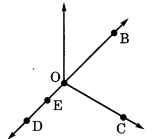Ex 4.1 Class 6 Maths Question 2.
Name the line given in all possible (twelve) ways, choosing only two letters at a times from the four given.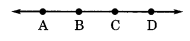Solution:
The given lines can be named as follows: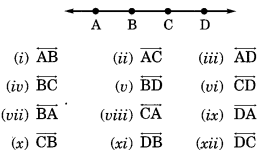Ex 4.1 Class 6 Maths Question 3.
Use the figure to name:
(a) Line containing point E.
(b) Line passing through A.
(c) Line on which 0 lies.
(d) Two pairs of intersecting lines.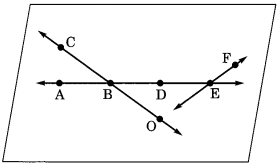Solution:Ex 4.1 Class 6 Maths Question 4.
How many lines can pass through
(a) one given point?
(b) two given points?
Solution:
(a) Infinitely many lines can pass through a given points.
(b) Only one line can pass through two given points.

Ex 4.1 Class 6 Maths Question 5.
Draw a rough figure and label suitably in each of the following cases:Solution:Ex 4.1 Class 6 Maths Question 6.
Consider the following figure of line MN. Say whether following statements are true or false in context of the given figure.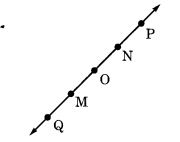Solution:
(a) True
(b) True
(c) True
(d) False
(e) False
(f) False
(g) True
(h) False
(i) False
(j) False
(k) True

## SabDekho

The Complete Educational Website